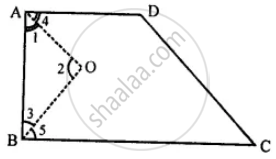# In a Quadrilateral Abcd, Ao and Bo Are Bisectors of Angle a and Angle B Respectively. Show That: - Mathematics

Sum

In a quadrilateral ABCD, AO and BO are bisectors of angle A and angle B respectively. Show that:

∠AOB = 1/2 (∠C + ∠D)

#### Solution

Given: AO and BO are the bisectors of ∠A and ∠B respectively.
∠1 = ∠4 and ∠3 = ∠5 ……..(i)To prove : ∠AOB = 1/2 (∠C + ∠D)

∠A + ∠B + ∠C + ∠D = 360°

1/2(∠A + ∠B + ∠C + ∠D) = 180° …………(ii)

Now in ∆AOB

∠1 + ∠2 + ∠3 = 180° ………(iii)

Equating equation (ii) and equation (iii), we get

∠1 + ∠2 + ∠3 = ∠A + ∠B + 1/2 (∠C + ∠D)

∠1 + ∠2 + ∠3 = ∠1 + ∠3 +1/2 (∠C + ∠D)

∠2 = 1/2 (∠C + ∠D)

∠AOB = 1/2 (∠C + ∠D)

Hence proved.

Is there an error in this question or solution?
Chapter 16: Understanding Shapes - Exercise 16 (C) [Page 188]

#### APPEARS IN

Selina Concise Mathematics Class 8 ICSE
Chapter 16 Understanding Shapes
Exercise 16 (C) | Q 15 | Page 188
Share